# 打造工业级推荐系统（十一）：基于内容的推荐算法## 二、基于内容的推荐算法实现原理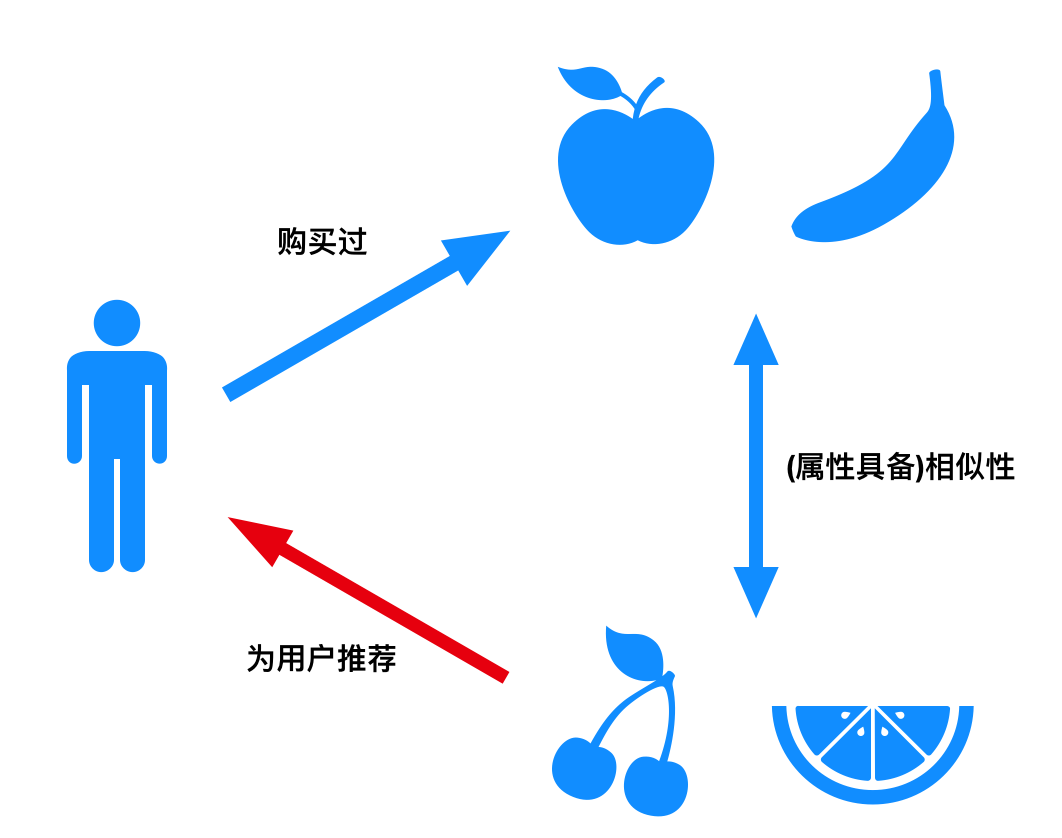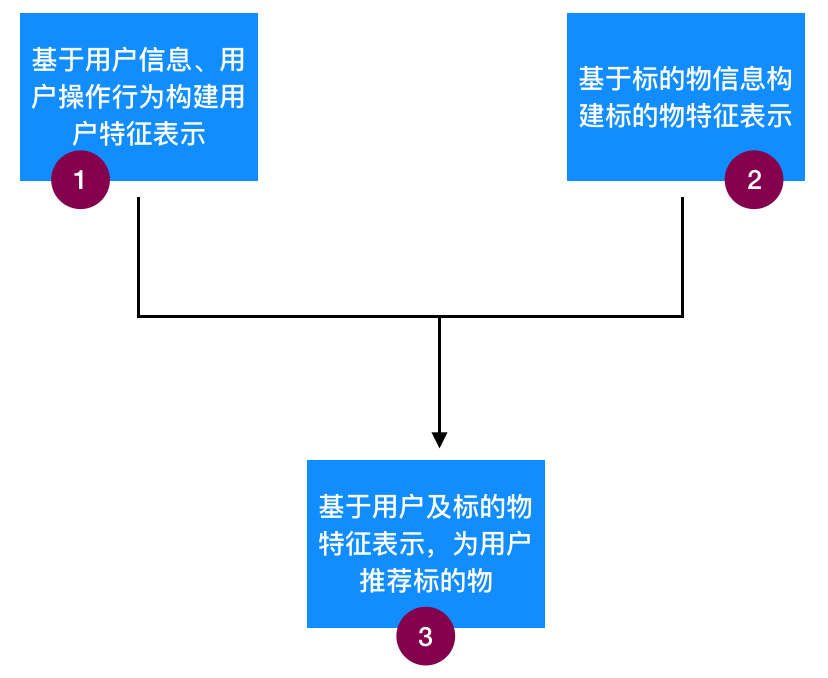### 3. 构建标的物特征表示

#### （1）标的物包含标签信息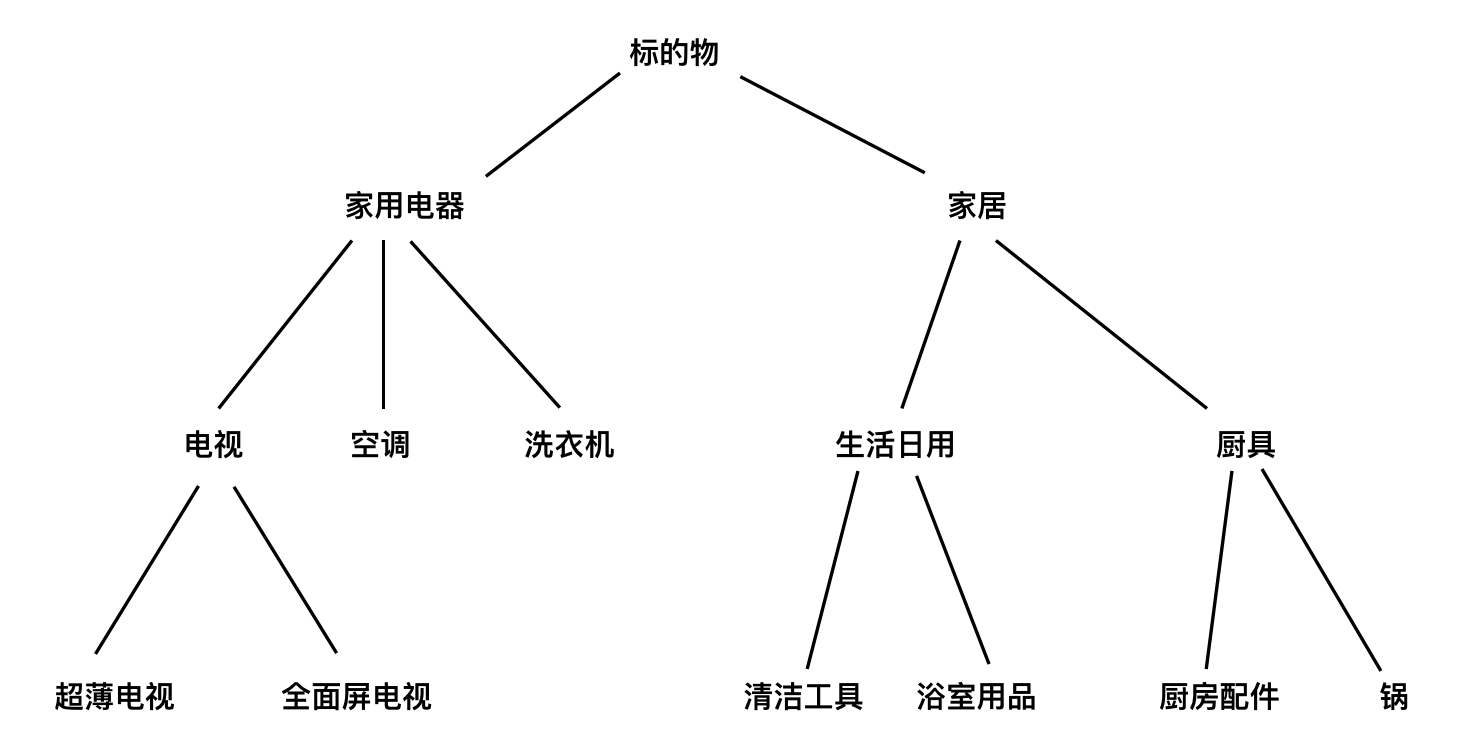#### （2）标的物具备结构化的信息

$V1=\left({p}_{1},{p}_{2},{p}_{3},\cdots \cdots ,{p}_{k}\right),V2=\left({q}_{1},{q}_{2},{q}_{3},\cdots \cdots ,{q}_{k}\right)$$V1=(p_1,p_2,p_3,\cdots\cdots,p_k),V2=(q_1,q_2,q_3,\cdots\cdots,q_k)$

$sim\left(V1,V2\right)\sum _{t=1}^{k}sim\left({p}_{t},{q}_{t}\right)$$sim(V1,V2)\sum\limits^k_{t=1}sim(p_t,q_t)$

$sim\left(V1,V2\right)=\sum _{t=1}^{k}{w}_{t}\ast sim\left({p}_{t},{q}_{t}\right)$$sim(V1,V2)=\sum\limits^k_{t=1}w_t*sim(p_t,q_t)$

#### （3）包含文本信息的标的物的特征表示

a. 利用 TF-IDF 将文本信息转化为特征向量

TF-IDF 通过将所有文档 (即标的物) 分词，获得所有不同词的集合 (假设有 M 个词)，那么就可以为每个文档构建一个 M 维 (每个词就是一个维度) 的向量，而该向量中某个词所在维度的值可以通过统计每个词在文档中的重要性来衡量，这个重要性的度量就是 TF-IDF。下面我们来详细说明 TF-IDF 是怎么计算的。

TF 即某个词在某篇文档中出现的频次，用于衡量这个词在文档中的重要性，出现次数越多的词重要性越大，当然我们会提前将“的”、“地”、“啊”等停用词去掉，这些词对构建向量是没有任何实际价值的，甚至是有害的。TF 具体计算公式如下，${t}_{k}$$t_k$ 是第 k 个词，${d}_{j}$$d_j$ 是第 j 个文档，下式中分子是 ${t}_{k}$$t_k$${d}_{j}$$d_j$ 中出现的次数，分母是 ${d}_{j}$$d_j$ 中词的总个数。

$TF\left({t}_{k},{d}_{j}\right)=\frac{|{t}_{k}\in {d}_{j}|}{|{d}_{j}|}$$TF(t_k,d_j)={|t_k\in d_j|\over|d_j|}$

IDF 代表的是某个词在所有文档中的“区分度”，如果某个词只在少量几个文档中出现，那么它包含的价值就是巨大的 (所谓物以稀为贵)，如果某个词在很多文档中出现，那么它就不能很好地衡量 (区分出) 这个文档。下面是 IDF 的计算公式，其中 N 是所有文档的个数，${n}_{k}$$n_k$ 是包含词 ${t}_{k}$$t_k$ 的文档个数，这个公式刚好跟前面的描述是一致的：稀有的词区分度大。

$IDF\left({t}_{k}\right)=\mathrm{log}\frac{N}{{n}_{k}}$$IDF(t_k)=\log {N\over n_k}$

$TF-IDF\left({t}_{k},{d}_{j}\right)=TF\left({t}_{k},{d}_{j}\right)\ast IDF\left({t}_{k}\right)$$TF-IDF(t_k,d_j)=TF(t_k,d_j)*IDF(t_k)$

b. 利用 LDA 算法构建文章 (标的物) 的主题

LDA 算法是一类文档主题生成模型，包含词、主题、文档三层结构，是一个三层的贝叶斯概率模型。 对于语料库中的每篇文档，LDA 定义了如下生成过程（generative process）：

 对每一篇文档，从主题分布中抽取一个主题；

 从上述被抽到的主题所对应的单词分布中抽取一个单词；

 重复上述过程直至遍历文档中的每一个单词。

c. 利用 doc2vec 算法构建文本相似度

doc2vec 或者叫做 paragraph2vec, sentence embeddings，是一种非监督式算法，可以获得 句子、段落、文章的稠密向量表达，它是 word2vec 的拓展，2014 年被 Google 的两位大牛提出，并大量用于文本分类和情感分析中。通过 doc2vec 学出句子、段落、文章的向量表示，可以通过计算向量之间距离来表达句子、段落、文章之间的相似性。

word2vec 通过学习一个唯一的向量表示每个词，每个词向量作为矩阵 W 中的一列 (W 是所有词的词向量构成的矩阵)，矩阵列可以通过词汇表为每个词做索引，排在索引第一位的放到矩阵 W 的第一列，如此类推。将学习问题转化为通过上下文词序列中前几个词来预测下一个词。具体的模型框架如下图：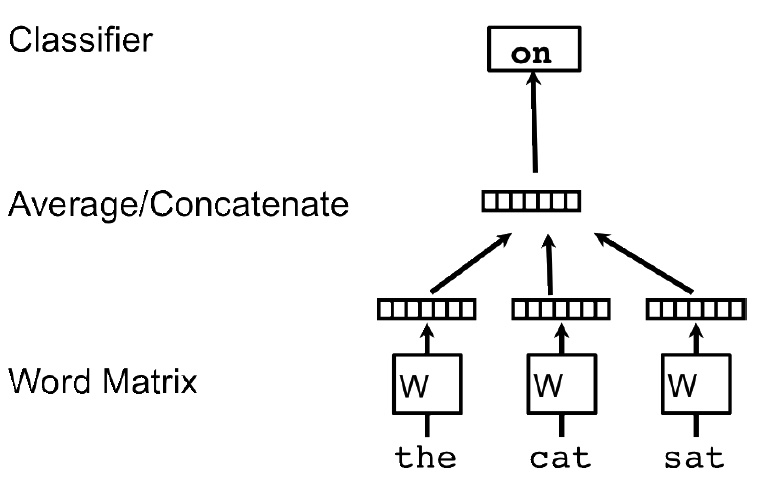${1\over T}\sum\limits^{T-k}{t=k}\log p(w_t | w{t-k},\dots,w_{t+k})$

$p\left({w}_{k}|{w}_{t-k},\dots ,{w}_{t+k}\right)=\frac{{e}^{{y}_{{w}_{t}}}}{{\sum }_{i}{e}^{{y}_{i}}}$$p(w_k|w_{t-k},\dots,w_{t+k})={e^{y_{w_t}}\over\sum\nolimits_ie^{y_i}}$

$y=b+Uh\left({w}_{t-k},\dots ,{w}_{t+k};W\right)$$y=b+Uh(w_{t-k},\dots,w_{t+k};W)$

word2vec 算法随机初始化词向量，通过随机梯度下降法来训练神经网络模型，最终得到每个词的向量表示。

doc2vec 类似地，每个段落 / 文档表示为向量，作为矩阵 D 的一列，每个词也表示为一个向量，作为矩阵 W 中的一列。将学习问题转化为通过上下文词序列中前几个词和段落 / 文档来预测下一个词。将段落 / 文档和词向量通过拼接或者平均来预测句子的下一个词 (下图是通过“the”、“cat”、“sat”及段落 id 来预测下一个词“on”)。在训练的时候我们固定上下文的长度，用滑动窗口的方法产生训练集。段落向量 / 句向量 在上下文中共享。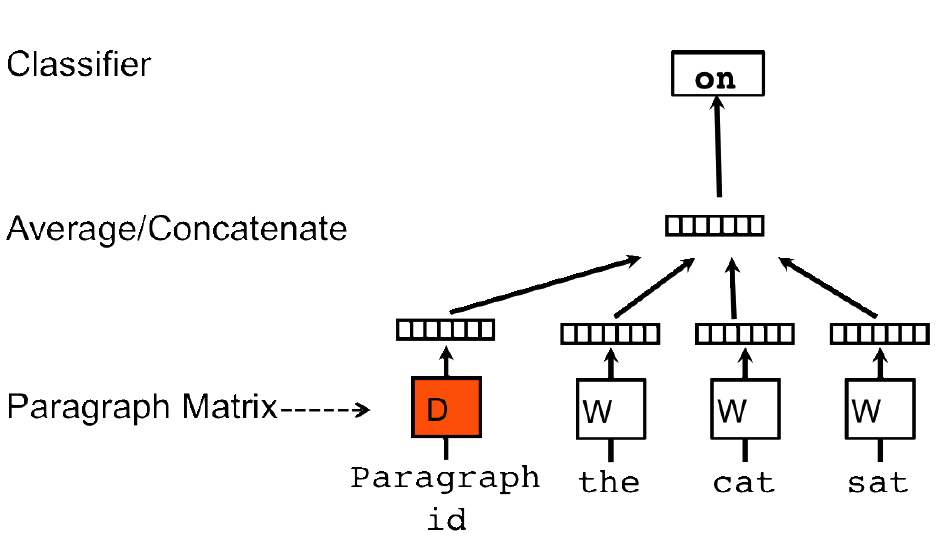#### （4）图片、音频、或者视频信息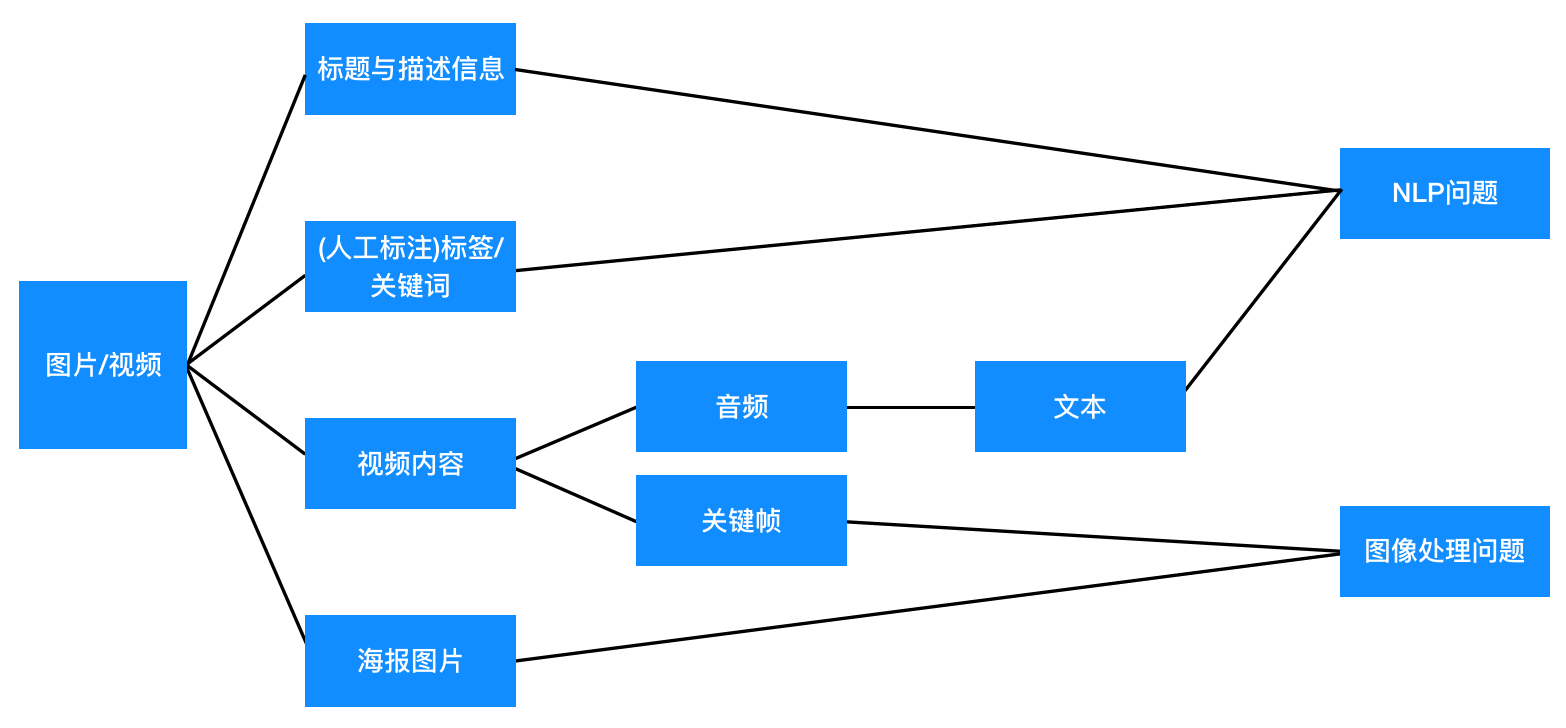### 4. 为用户做个性化推荐

#### （1）采用跟基于物品的协同过滤类似的方式推荐

$sim\left(u,s\right)=su{m}_{{s}_{i}\in S}score\left(u,{s}_{i}\right)\ast sim\left({s}_{i},s\right)$$sim(u,s)=sum_{s_i\in S}score(u,s_i)*sim(s_i,s)$

$Rec\left(u\right)=su{m}_{{s}_{i}\in U}{s}_{j}|{s}_{j}\in kNN\left({s}_{i}\right)$$Rec(u)=sum_{s_i\in U}{s_j|s_j\in kNN(s_i)}$

## （2）采用跟基于用户协同过滤类似的方法计算推荐

$sim\left(u,s\right)=\sum _{{u}_{i}\in U}sim\left(u,{u}_{i}\right)\ast score\left({u}_{i},s\right)$$sim(u,s)=\sum_{u_i\in U}sim(u,u_i)*score(u_i,s)$

$Rec\left(u\right)=\sum _{{u}_{i}\in kNN\left(u\right)}{s}_{j}\in A\left({u}_{i}\right)$$Rec(u)=\sum_{u_i\in kNN(u)}{s_j\in A(u_i)}$

#### （3）基于标的物聚类的推荐

$Rec(u)=\sum\limits_{s\in H}{t\in Cluster(s)~&~t\neq s}$

#### （4）基于向量相似的推荐

FAISS 库适合稠密向量的检索和聚类，所以对于利用 LDA、Doc2vector 算法构建向量表示的方案是实用的，因为这些方法构建的是稠密向量。而对于 TF-IDF 及基于标签构建的向量化表示，就不适用了，这两类方法构建的都是稀疏的高维向量。

#### （5）基于标签的反向倒排索引做推荐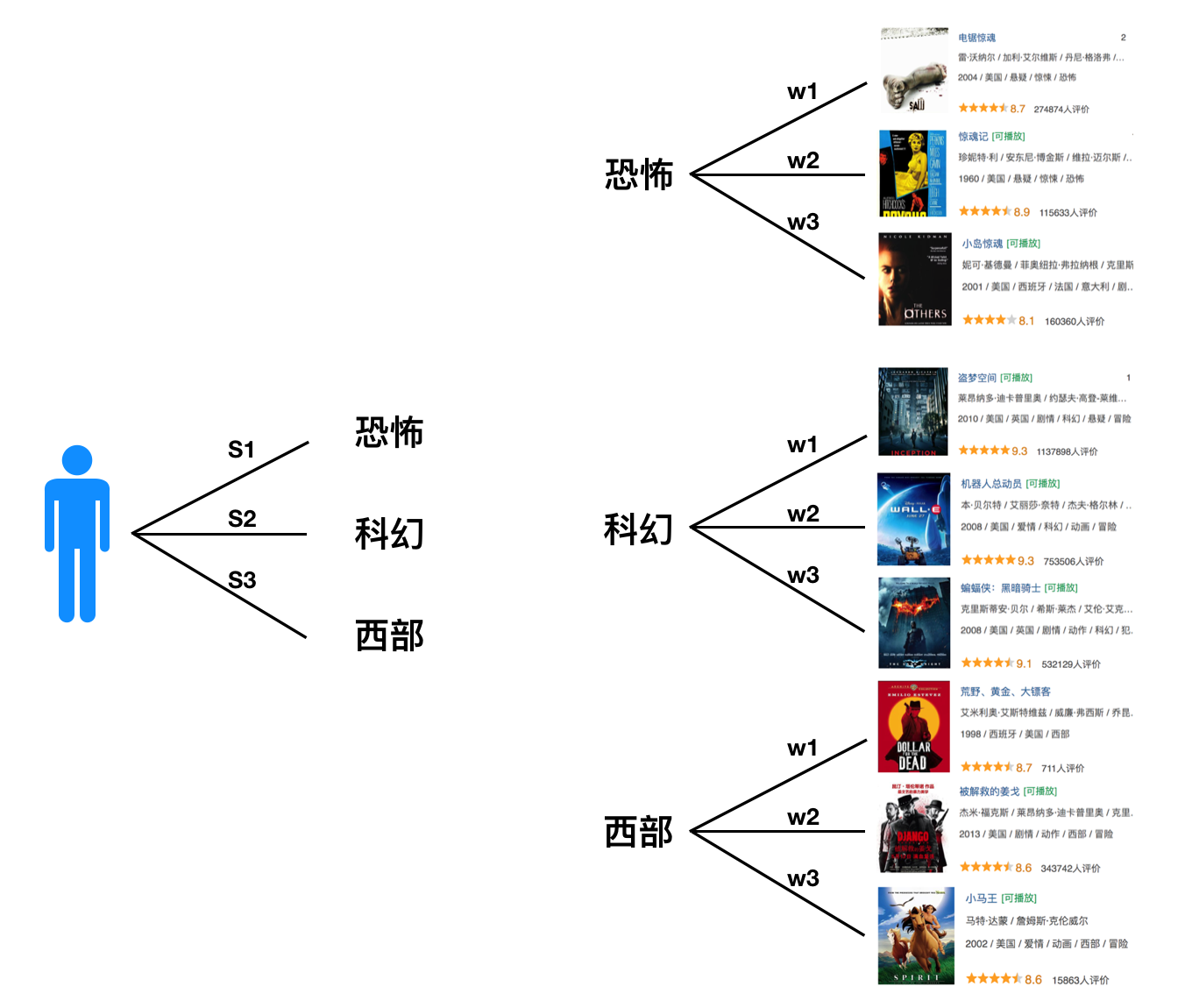$\left({T}_{1},{S}_{1}\right),\left({T}_{2},{S}_{2}\right),\left({T}_{3},{S}_{3}\right),\dots ,\left({T}_{k},{S}_{k}\right)$${(T_1,S_1),(T_2,S_2),(T_3,S_3),\dots,(T_k,S_k)}$

$\begin{array}{rl}& {T}_{1}↔\left({O}_{11},{w}_{11}\right),\left({O}_{12},{w}_{12}\right),\left({O}_{13},{w}_{13}\right),\dots ,\left({O}_{1{p}_{1}},{w}_{1{p}_{1}}\right)\mathrm{&}{T}_{2}↔\left({O}_{21},{w}_{21}\right),\left({O}_{22},{w}_{22}\right),\left({O}_{23},{w}_{23}\right),\dots ,\left({O}_{2{p}_{2}},{w}_{2{p}_{2}}\right)\mathrm{&}\dots \dots \mathrm{&}{T}_{k}↔\left({O}_{k1},{w}_{k1}\right),\left({O}_{k2},{w}_{k2}\right),\left({O}_{k3},{w}_{k3}\right),\dots ,\left({O}_{k{p}_{k}},{w}_{k{p}_{k}}\right)\end{array}$\begin{aligned}&T_1\leftrightarrow{(O_{11},w_{11}),(O_{12},w_{12}),(O_{13},w_{13}),\dots,(O_{1p_1},w_{1p_1})}\&T_2\leftrightarrow{(O_{21},w_{21}),(O_{22},w_{22}),(O_{23},w_{23}),\dots,(O_{2p_2},w_{2p_2})}\&\dots\dots\&T_k\leftrightarrow{(O_{k1},w_{k1}),(O_{k2},w_{k2}),(O_{k3},w_{k3}),\dots,(O_{kp_k},w_{kp_k})}\end{aligned}

\begin{aligned}U&=\sum^k_{i=1}S_iT_i\&=\sum^k_{i=1}S_i{(O_{i1},w_{i1}),(O_{i2},w_{i2}),\dots,(O_{ip_i},w_{ip_i})}\&=\sum^k_{i=1}\sum^{p_i}{j=1}S_i*w{ij}*O_{ij}\end{aligned}

## 三、基于内容的推荐算法应用场景

### 4. 主题推荐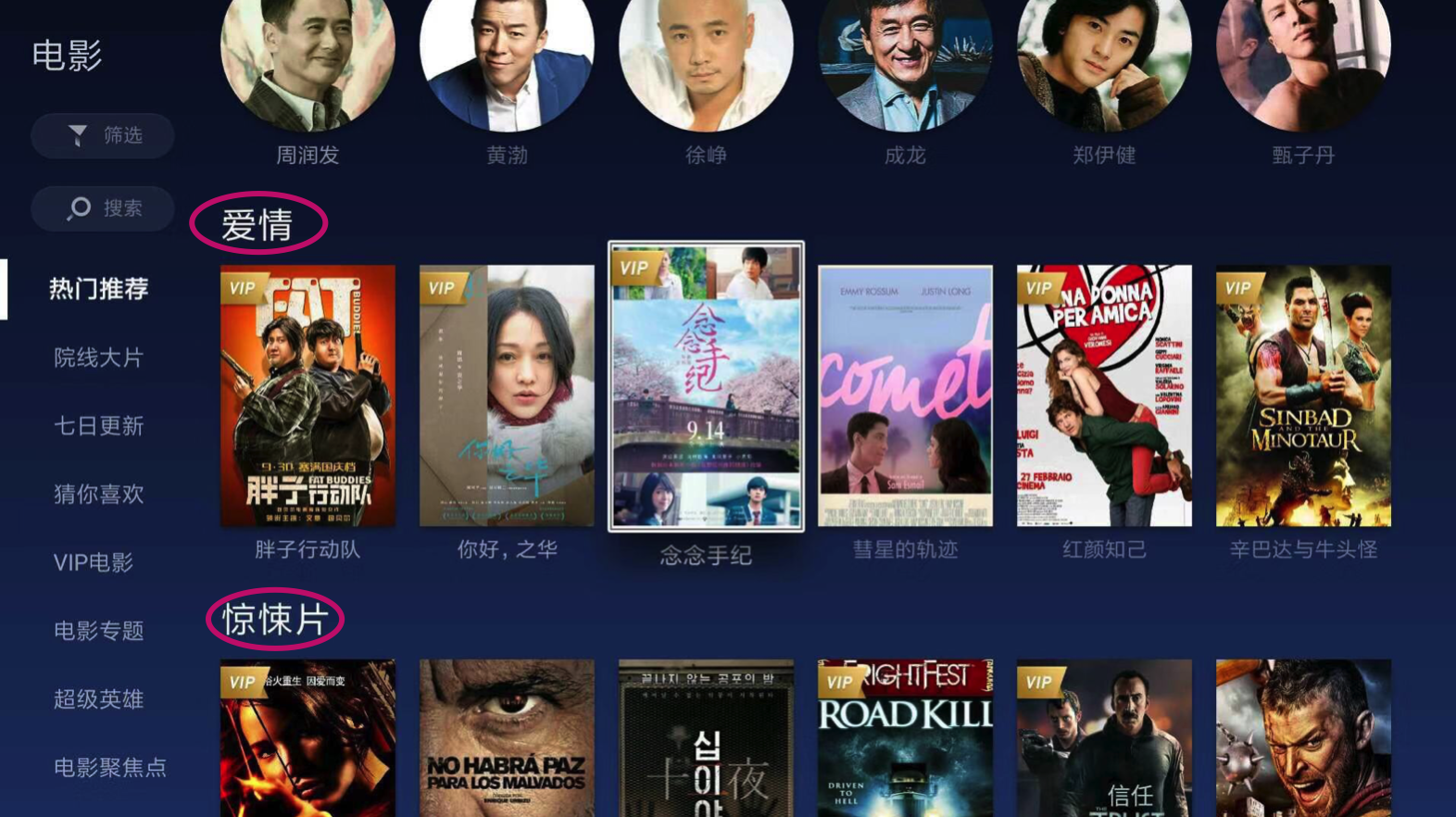## 五、算法落地需要关注的重要问题

### 2. 怎么利用负反馈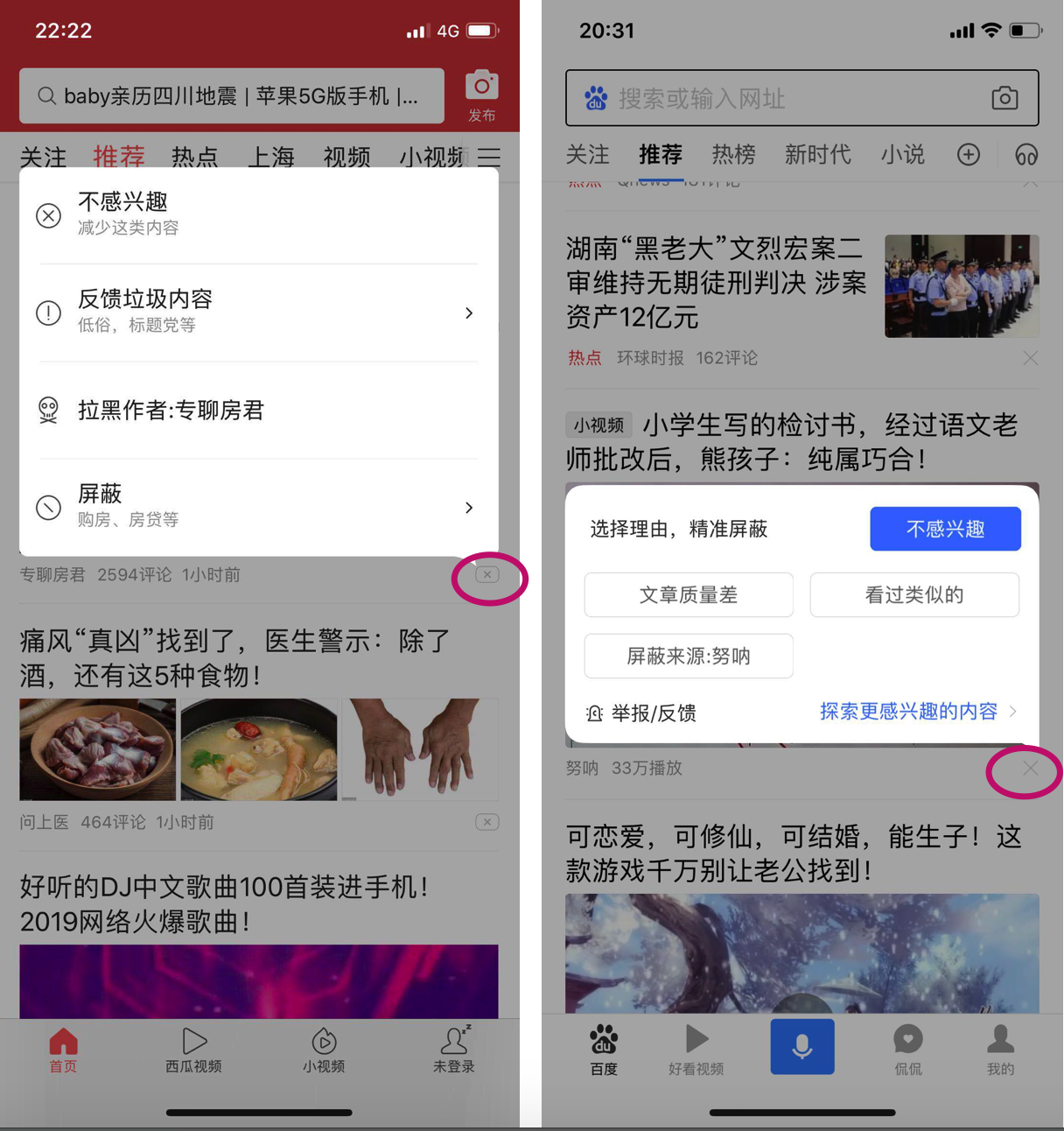## 写在最后

1. Latent Dirichlet Allocation
2. LightLDA- Big Topic Models on Modest Computer Clusters
3. Efficient Estimation of Word Representations in Vector Space
4. Distributed Representations of Words and Phrases and their Compositionality
5. Distributed Representations of Sentences and Documents
6. Content-based recommender systems: State of the art and trends
7. Content-based collaborative recommendation
8.  Content-based recommendation systems